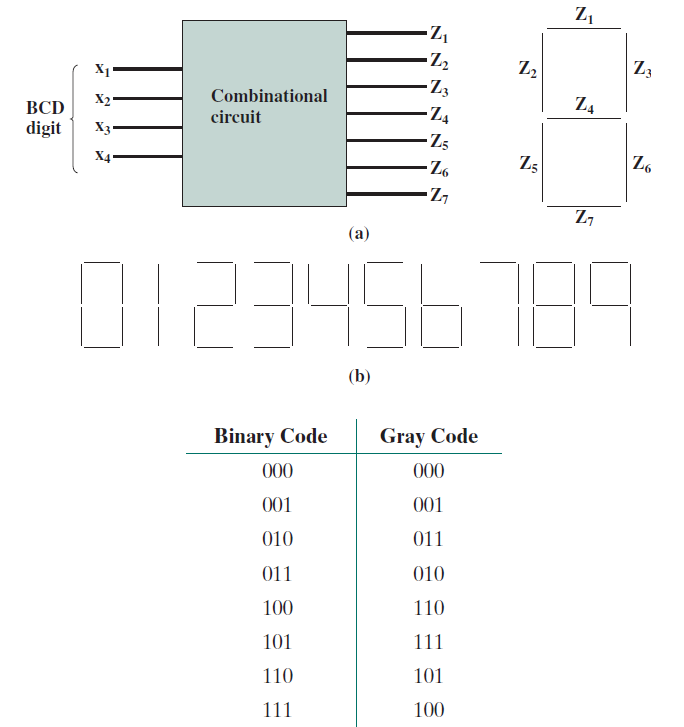Home / Expert Answers / Electrical Engineering / the-gray-code-is-a-binary-code-for-integers-it-differs-from-the-ordinary-binary-representation-in-t-pa262

# (Solved): The Gray code is a binary code for integers. It differs from the ordinary binary representation in t ...

The Gray code is a binary code for integers. It differs from the ordinary binary representation in that there is just a single bit change between the representations of any two numbers. This is useful for applications such as counters or analog-to-digital converters where a sequence of numbers is generated. Because only one bit changes at a time, there is never any ambiguity due to slight timing differences. The first eight elements of the code are1. Design a circuit with Logisim that converts from binary to 7-segment display decimal - a).

2. Design a circuit with Logisim that converts from binary to Gray code.

(b)

We have an Answer from Expert